# 2.7 Discussion and Exercises

Most of the data structures described in this chapter are folklore. They can be found in implementations dating back over 30 years. For example, implementations of stacks, queues, and deques, which generalize easily to the ArrayStack, ArrayQueue and ArrayDeque structures described here, are discussed by Knuth [46, Section 2.2.2].

Brodnik et al.  seem to have been the first to describe the RootishArrayStack and prove a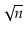lower-bound like that in Section 2.6.2. They also present a different structure that uses a more sophisticated choice of block sizes in order to avoid computing square roots in the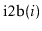method. Within their scheme, the block containing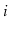is block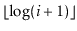, which is simply the index of the leading 1 bit in the binary representation of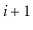. Some computer architectures provide an instruction for computing the index of the leading 1-bit in an integer.

A structure related to the RootishArrayStack is the two-level tiered-vector of Goodrich and Kloss . This structure supports the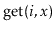andoperations in constant time and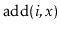and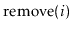in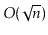time. These running times are similar to what can be achieved with the more careful implementation of a RootishArrayStack discussed in Exercise 2.10.

Exercise 2..1   The List method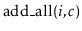inserts all elements of the Collection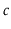into the list at position. (Themethod is a special case where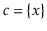.) Explain why, for the data structures in this chapter, it is not efficient to implementby repeated calls to. Design and implement a more efficient implementation.

Exercise 2..2   Design and implement a RandomQueue. This is an implementation of the Queue interface in which the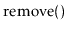operation removes an element that is chosen uniformly at random among all the elements currently in the queue. (Think of a RandomQueue as a bag in which we can add elements or reach in and blindly remove some random element.) Theandoperations in a RandomQueue should run in constant time per operation.

Exercise 2..3   Design and implement a Treque (triple-ended queue). This is a List implementation in which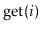and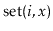run in constant time andandrun in timeIn other words, modifications are fast if they are near either end or near the middle of the list.

Exercise 2..4   Implement a method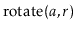that rotates'' the array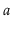so that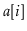moves to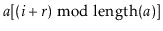, for all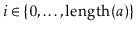.

Exercise 2..5   Implement a method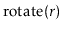that rotates'' a List so that list itembecomes list item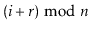. When run on an ArrayDeque, or a DualArrayDeque,should run in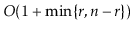time.

Exercise 2..6   This exercise is left out of the pseudocode edition.

Exercise 2..7   Modify the ArrayDeque implementation so that it does not use the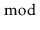operator (which is expensive on some systems). Instead, it should make use of the fact that, if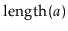is a power of 2, then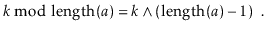(Here,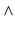is the bitwise-and operator.)

Exercise 2..8   Design and implement a variant of ArrayDeque that does not do any modular arithmetic at all. Instead, all the data sits in a consecutive block, in order, inside an array. When the data overruns the beginning or the end of this array, a modified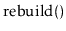operation is performed. The amortized cost of all operations should be the same as in an ArrayDeque.

Hint: Getting this to work is really all about how you implement theoperation. You would liketo put the data structure into a state where the data cannot run off either end until at least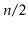operations have been performed.

Test the performance of your implementation against the ArrayDeque. Optimize your implementation (by using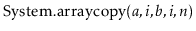) and see if you can get it to outperform the ArrayDeque implementation.

Exercise 2..9   Design and implement a version of a RootishArrayStack that has onlywasted space, but that can performandoperations in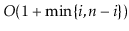time.

Exercise 2..10   Design and implement a version of a RootishArrayStack that has onlywasted space, but that can performand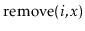operations intime. (For an idea on how to do this, see Section 3.3.)

Exercise 2..11   Design and implement a version of a RootishArrayStack that has onlywasted space, but that can performandoperations in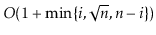time. (See Section 3.3 for ideas on how to achieve this.)

Exercise 2..12   Design and implement a CubishArrayStack. This three level structure implements the List interface usingwasted space. In this structure,andtake constant time; whileandtake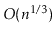amortized time.

opendatastructures.org